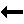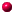What is this thing called Painlevé''?ordinary points     Regular Singular Points and Movable Singularities Recall that we are focussing on scalar ODEs governing a function $$y(x)$$ of a complex variable $$x$$ such as $$y^{(n)}=F(y^{(n-1)}, ..., y', y, x), \quad (0.3)$$ where $$F$$ is rational in $$y^{(n-1)}, ..., y', y$$ and analytic in $$x$$ except at a finite number of isolated points $$x_i$$, $$i=0, ..., s-1$$ where $$s$$ is a nonnegative integer. Definition: Suppose $$F$$ is linear in $$y^{(n-1)}, ..., y', y$$: $$F=G_{n-1}y^{(n-1)}+ ... + G_0 y,$$ where $$G_k$$ are functions of $$x$$ at least one of which is not analytic at $$x=x_0$$. Then $$x_0$$ is a regular singular point if $$(x-x_0)^{n-k}G_k, k=0, ..., n-1$$ are all analytic at $$x=x_0$$. Consider the example (compare this with Equation (0.2) in Section 0.1) $$y'=- y/x \qquad\qquad (0.4)$$ Clearly $$x_0=0$$ is a regular singular point. The general solution is $$y=c/x$$.Back to ContentsNalini Joshi Last modified: 12 October 2011 by N.Joshi